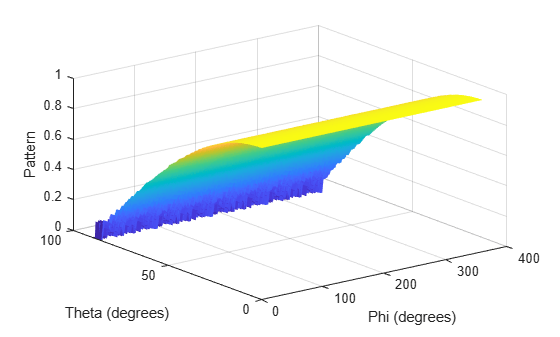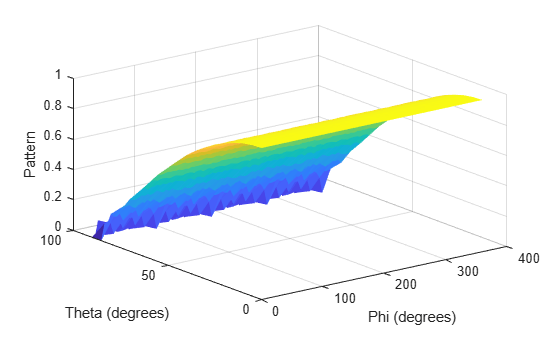Documentation

# uv2phithetapat

Convert radiation pattern from u/v form to phi/theta form

## Syntax

``pat_phitheta = uv2phithetapat(pat_uv,u,v)``
``pat_phitheta = uv2phithetapat(pat_uv,u,v,phi,theta)``
``````[pat_phitheta,phi_pat,theta_pat] = uv2phithetapat(___)``````

## Description

example

````pat_phitheta = uv2phithetapat(pat_uv,u,v)` expresses the antenna radiation pattern `pat_phitheta` in φ/θ angle coordinates instead of u/v space coordinates. `pat_uv` samples the pattern at u angles in `u` and v angles in `v`. The `pat_phitheta` matrix uses a default grid that covers φ values from 0 to 360 degrees and θ values from 0 to 90 degrees. In this grid, `pat_phitheta` is uniformly sampled with a step size of 1 for φ and θ. The function interpolates to estimate the response of the antenna at a given direction.```

example

````pat_phitheta = uv2phithetapat(pat_uv,u,v,phi,theta)` uses vectors `phi` and `theta` to specify the grid at which to sample `pat_phitheta`. To avoid interpolation errors, `phi` should cover the range [0, 360], and `theta` should cover the range [0, 90].```

example

``````[pat_phitheta,phi_pat,theta_pat] = uv2phithetapat(___)``` returns vectors containing the φ and θ angles at which `pat_phitheta` samples the pattern, using any of the input arguments in the previous syntaxes.```

## Examples

collapse all

Convert a radiation pattern to φ-θ space with the angles spaced 1° apart.

Define the pattern in terms of u and v. Because u and v values outside the unit circle are not physical, set the pattern values in this region to zero.

```u = -1:0.01:1; v = -1:0.01:1; [u_grid,v_grid] = meshgrid(u,v); pat_uv = sqrt(1 - u_grid.^2 - v_grid.^2); pat_uv(hypot(u_grid,v_grid) >= 1) = 0;```

Convert the pattern to φ-θ space.

`[pat_phitheta,phi,theta] = uv2phithetapat(pat_uv,u,v);`

Convert a radiation pattern to $\varphi -\theta$ space with the angles spaced one degree apart.

Define the pattern in terms of $u$ and $v$. For values outside the unit circle, $u$ and $v$ are undefined, and the pattern value is 0.

```u = -1:0.01:1; v = -1:0.01:1; [u_grid,v_grid] = meshgrid(u,v); pat_uv = sqrt(1 - u_grid.^2 - v_grid.^2); pat_uv(hypot(u_grid,v_grid) >= 1) = 0;```

Convert the pattern to $\varphi -\theta$ space. Store the $\varphi$ and $\theta$ angles for use in plotting.

`[pat_phitheta,phi,theta] = uv2phithetapat(pat_uv,u,v);`

Plot the result.

```H = surf(phi,theta,pat_phitheta); H.LineStyle = 'none'; xlabel('Phi (degrees)'); ylabel('Theta (degrees)'); zlabel('Pattern');```Convert a radiation pattern to $\varphi -\theta$ space with the angles spaced five degrees apart.

Define the pattern in terms of $u$ and $v$. For values outside the unit circle, $u$ and $v$ are undefined, and the pattern value is 0.

```u = -1:0.01:1; v = -1:0.01:1; [u_grid,v_grid] = meshgrid(u,v); pat_uv = sqrt(1 - u_grid.^2 - v_grid.^2); pat_uv(hypot(u_grid,v_grid) >= 1) = 0;```

Define the set of $\varphi$ and $\theta$ angles at which to sample the pattern. Then, convert the pattern.

```phi = 0:5:360; theta = 0:5:90; pat_phitheta = uv2phithetapat(pat_uv,u,v,phi,theta);```

Plot the result.

```H = surf(phi,theta,pat_phitheta); H.LineStyle = 'none'; xlabel('Phi (degrees)'); ylabel('Theta (degrees)'); zlabel('Pattern');```## Input Arguments

collapse all

Antenna radiation pattern in u/v form, specified as a Q-by-P matrix. `pat_uv` samples the 3-D magnitude pattern in decibels, in terms of u and v coordinates. P is the length of the `u` vector, and Q is the length of the `v` vector.

Data Types: `double`

u coordinates at which `pat_uv` samples the pattern, specified as a vector of length P. Each coordinate is between –1 and 1.

Data Types: `double`

v coordinates at which `pat_uv` samples the pattern, specified as a vector of length Q. Each coordinate is between –1 and 1.

Data Types: `double`

Phi angles at which `pat_phitheta` samples the pattern, specified as a vector of length L. Each φ angle is in degrees, between 0 and 360.

Data Types: `double`

Theta angles at which `pat_phitheta` samples the pattern, specified as a vector of length M. Each θ angle is in degrees, between 0 and 90. Such θ angles are in the hemisphere for which u and v are defined.

Data Types: `double`

## Output Arguments

collapse all

Antenna radiation pattern in phi/theta form, returned as an M-by-L matrix. `pat_phitheta` samples the 3-D magnitude pattern in decibels, in terms of φ and θ angles. L is the length of the `phi_pat` vector, and M is the length of the `theta` vector.

Phi angles at which `pat_phitheta` samples the pattern, returned as a vector of length L. Angles are expressed in degrees.

Theta angles at which `pat_phitheta` samples the pattern, returned as a vector of length M. Angles are expressed in degrees.

## More About

collapse all

### U/V Space

The u and v coordinates are the direction cosines of a vector with respect to the y-axis and z-axis, respectively.

The u/v coordinates for the hemisphere x ≥ 0 are derived from the phi and theta angles, as follows:

`$\begin{array}{l}u=\mathrm{sin}\theta \mathrm{cos}\varphi \\ v=\mathrm{sin}\theta \mathrm{sin}\varphi \end{array}$`

In these expressions, φ and θ are the phi and theta angles, respectively.

In terms of azimuth and elevation, the u and v coordinates are

`$\begin{array}{l}u=\mathrm{cos}el\mathrm{sin}az\\ v=\mathrm{sin}el\end{array}$`

The values of u and v satisfy the inequalities

`$\begin{array}{l}-1\le u\le 1\\ -1\le v\le 1\\ {u}^{2}+{v}^{2}\le 1\end{array}$`

Conversely, the phi and theta angles can be written in terms of u and v using

`$\begin{array}{l}\mathrm{tan}\varphi =u/v\\ \mathrm{sin}\theta =\sqrt{{u}^{2}+{v}^{2}}\end{array}$`

The azimuth and elevation angles can also be written in terms of u and v

`$\begin{array}{l}\mathrm{sin}el=v\\ \mathrm{tan}az=\frac{u}{\sqrt{1-{u}^{2}-{v}^{2}}}\end{array}$`

### Phi Angle, Theta Angle

The φ angle is the angle from the positive y-axis toward the positive z-axis, to the vector’s orthogonal projection onto the yz plane. The φ angle is between 0 and 360 degrees. The θ angle is the angle from the x-axis toward the yz plane, to the vector itself. The θ angle is between 0 and 180 degrees.

The figure illustrates φ and θ for a vector that appears as a green solid line. The coordinate system is relative to the center of a uniform linear array, whose elements appear as blue circles.The coordinate transformations between φ/θ and az/el are described by the following equations

`$\begin{array}{l}\mathrm{sin}\left(\text{el}\right)=\mathrm{sin}\varphi \mathrm{sin}\theta \hfill \\ \mathrm{tan}\left(\text{az}\right)=\mathrm{cos}\varphi \mathrm{tan}\theta \hfill \\ \hfill \\ \mathrm{cos}\theta =\mathrm{cos}\left(\text{el}\right)\mathrm{cos}\left(\text{az}\right)\hfill \\ \mathrm{tan}\varphi =\mathrm{tan}\left(\text{el}\right)/\mathrm{sin}\left(\text{az}\right)\hfill \end{array}$`

## See Also

### Topics

#### Hybrid Beamforming for Massive MIMO Phased Array Systems

Download the white paper